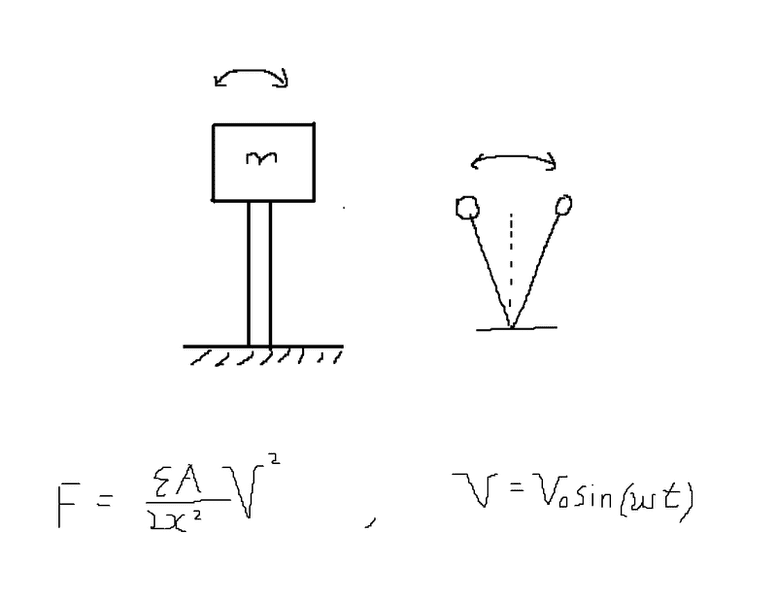# Resonant frequency of this pendulum?

unscientific
Hi everyone,

I'm planning a simple experiment for my school, which involves fixing a mass M onto a rod of length L made of a copper alloy.

Suppose I drive it capacitively, how do I find the resonant frequency of this simple oscillator? I need its resonant frequency to be about 400-500Hz, which would depend on it's geometry.

I know its:

-modulus of elasticity
-density
-Shear modulus
-torsion constantI have found that the force varies on sin2(ωt), so the drive frequency of the oscillator is the drive frequency of the voltage. I know that at resonance, the amplitude is approxiamtely

≈ Q(τ/k)

but what I need to find is its resonant frequency.

Last edited: